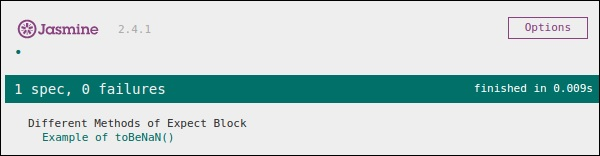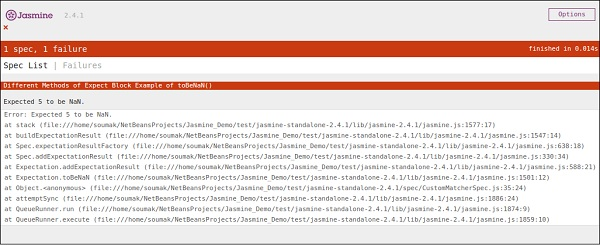# JasmineJS - Not a Number Check

Jasmine provides a special matcher to check this special type of testing scenario that is toBeNaN().

Let us modify our customerMatcher.js with the following code.

```describe("Different Methods of Expect Block",function () {
it("Example of toBeNaN()", function () {
expect(0 / 0).toBeNaN();
});
});
```

Here we want to test what is the value of “0/0” which cannot be determined. Hence, this piece of code will generate the following green screenshot.Now let us again modify the code with the following logic, where we will assign one variable exp to 25 and expect the result is not a number one dividing it with 5.

```describe("Different Methods of Expect Block",function () {
var exp = 25;

it("Example of toBeNaN()", function () {
expect(exp/5).toBeNaN();
});
});
```

This piece of code will yield the following output.